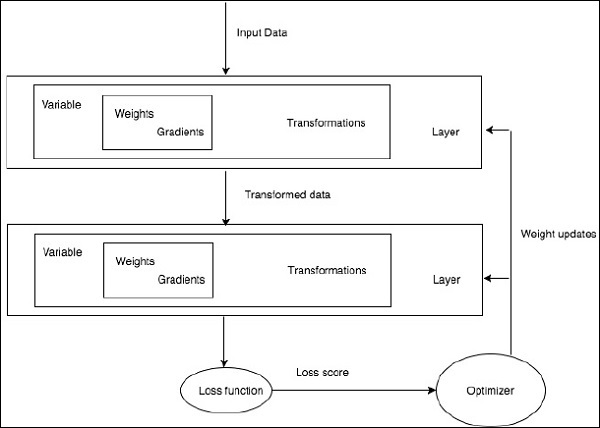# PyTorch - Neural Networks to Functional Blocks

Training a deep learning algorithm involves the following steps −

• Building a data pipeline
• Building a network architecture
• Evaluating the architecture using a loss function
• Optimizing the network architecture weights using an optimization algorithm

Training a specific deep learning algorithm is the exact requirement of converting a neural network to functional blocks as shown below −With respect to the above diagram, any deep learning algorithm involves getting the input data, building the respective architecture which includes a bunch of layers embedded in them.

If you observe the above diagram, the accuracy is evaluated using a loss function with respect to optimization of the weights of neural network.